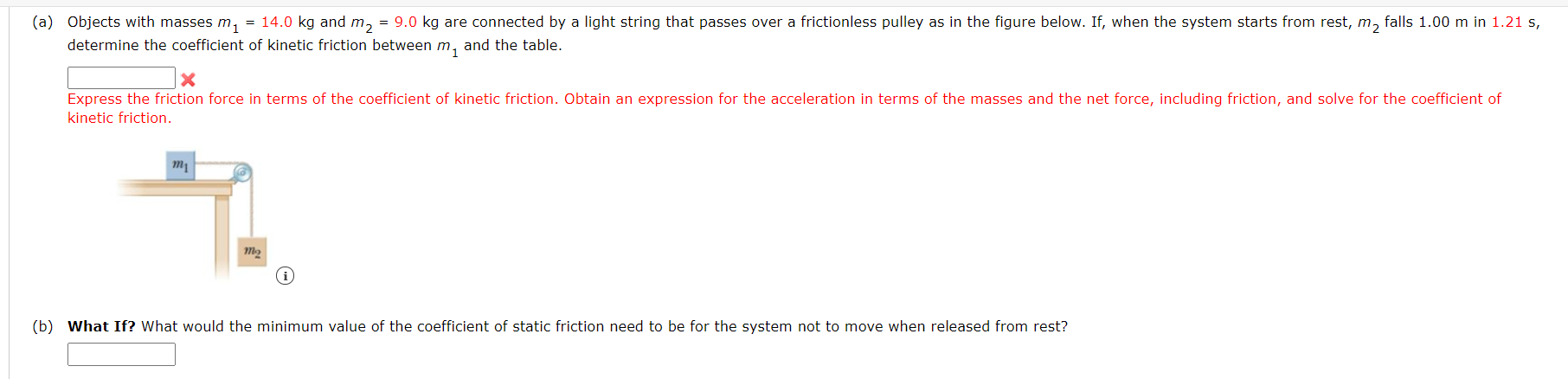# Question (a) Objects with masses m1 = 14.0 kg and m2 = 9.0 kg are connected by a light string that passes over a frictionless pulley as in the figure below. If, when the system starts from rest, m, falls 1.00 m in 1.21 s, determine the coefficient of kinetic friction between m, and the table. x Express the friction force in terms of the coefficient of kinetic friction. Obtain an expression for the acceleration in terms of the masses and the net force, including friction, and solve for the coefficient of kinetic friction. m2 i (b) What If? What would the minimum value of the coefficient of static friction need to be for the system not to move when released from rest?